Kindergarten
1st
2nd
3rd
4th
5th
6th
7th
8th
9th
10th
11th
12th
Higher Ed
Other
Subjects
ELA
Math
Science
Social Studies
Art
Computer Science
French
German
Music
Physical Education
Spanish
Other
Private Library
Algebra 1 8-5 Factoring x² + bx + c
starstarstarstarstarstarstarstarstarstar
by Narly Cabral-Nunez
| 27 Questions
The outlined content above was added from outside of Formative.
The outlined content above was added from outside of Formative.
zoom in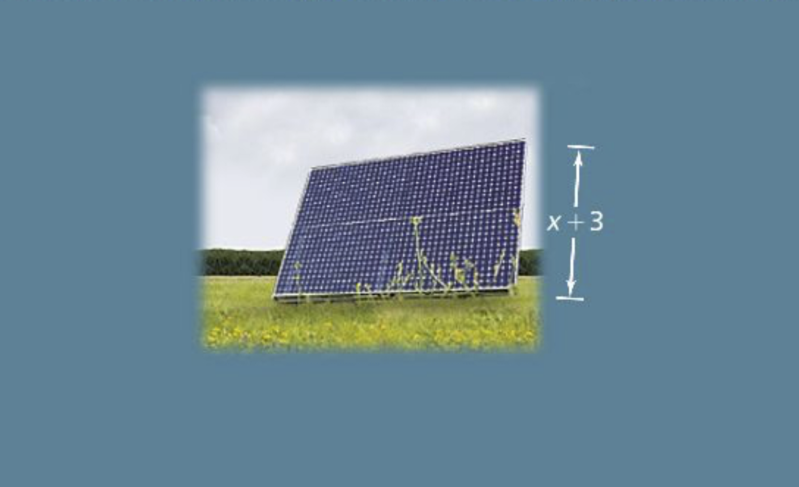1
10 pts
Solve It! The area of a rectangular solar panel is given by the trinomial below.
The height of the solar panel is given by the binomial below.
What is an expression for the length of the panel?The outlined content above was added from outside of Formative.
zoom in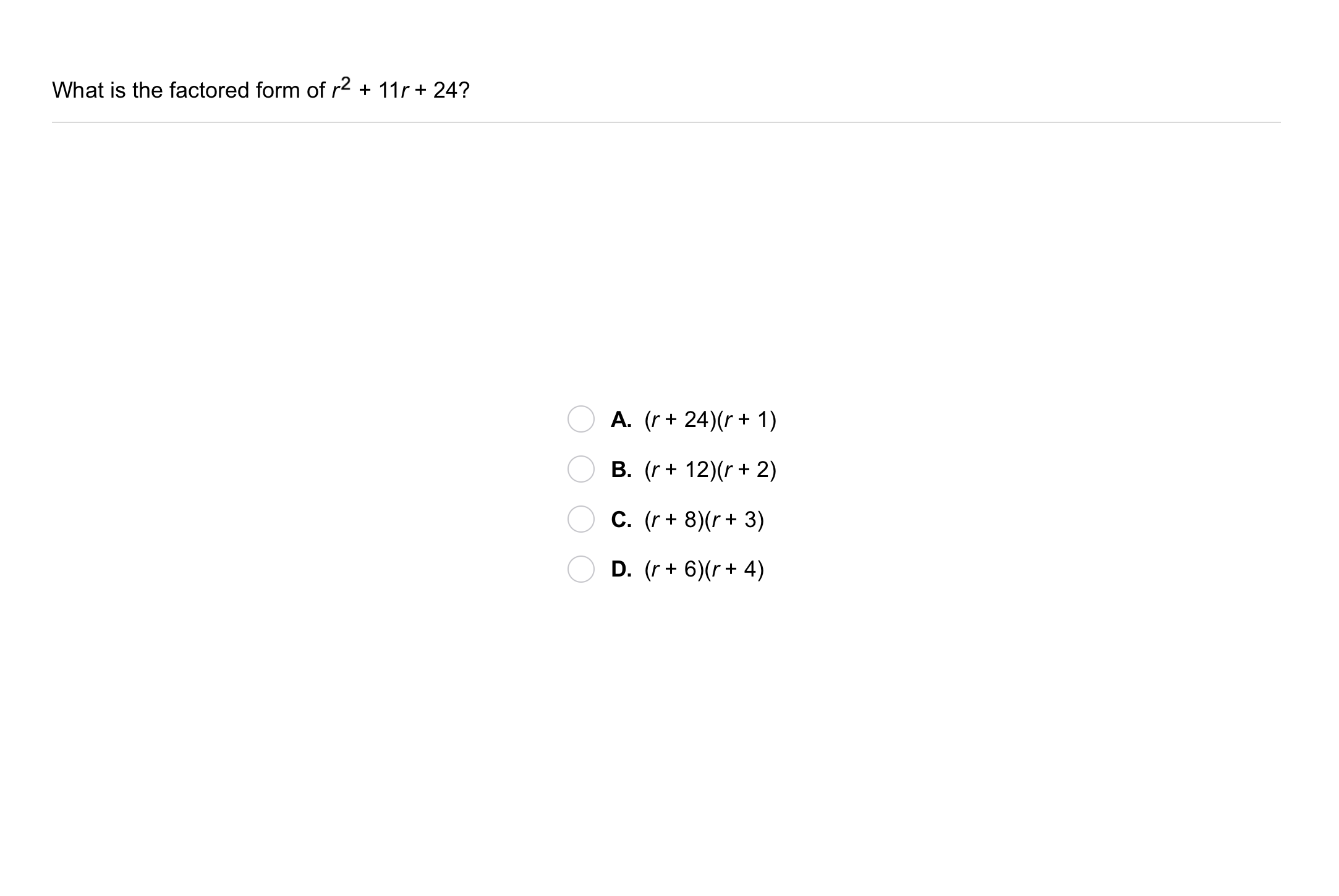2
10 pts
Problem 1 Got It?
A
B
C
D
The outlined content above was added from outside of Formative.
3
10 pts
Problem 2 Got It? What is the factored form of the expression?

The outlined content above was added from outside of Formative.
zoom in5
10 pts
Problem 3 Got It?
A
B
C
D
zoom in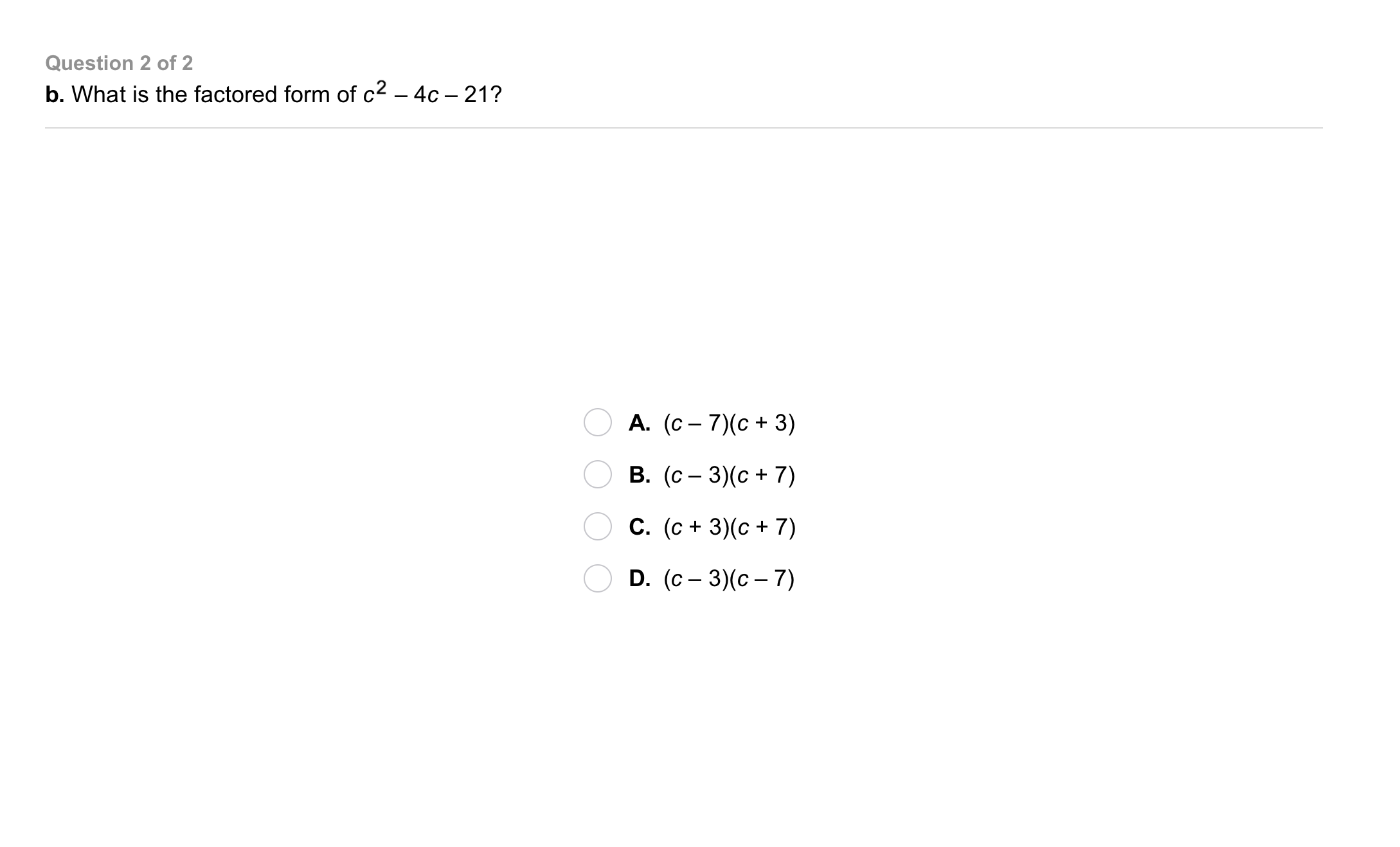6
10 pts
Problem 3 Got It?
A
B
C
D
The outlined content above was added from outside of Formative.
zoom in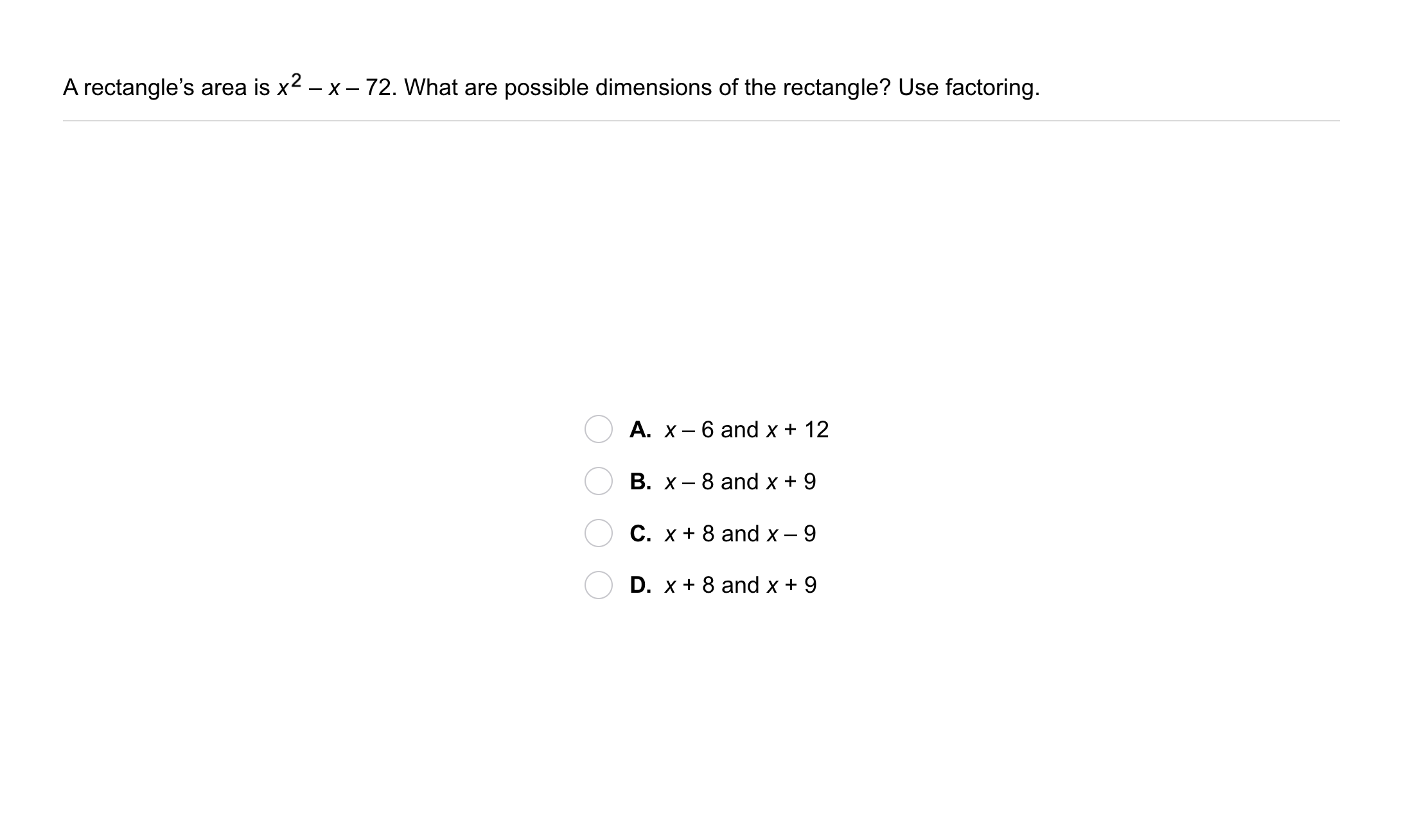7
10 pts
Problem 4 Got It?
A
B
C
D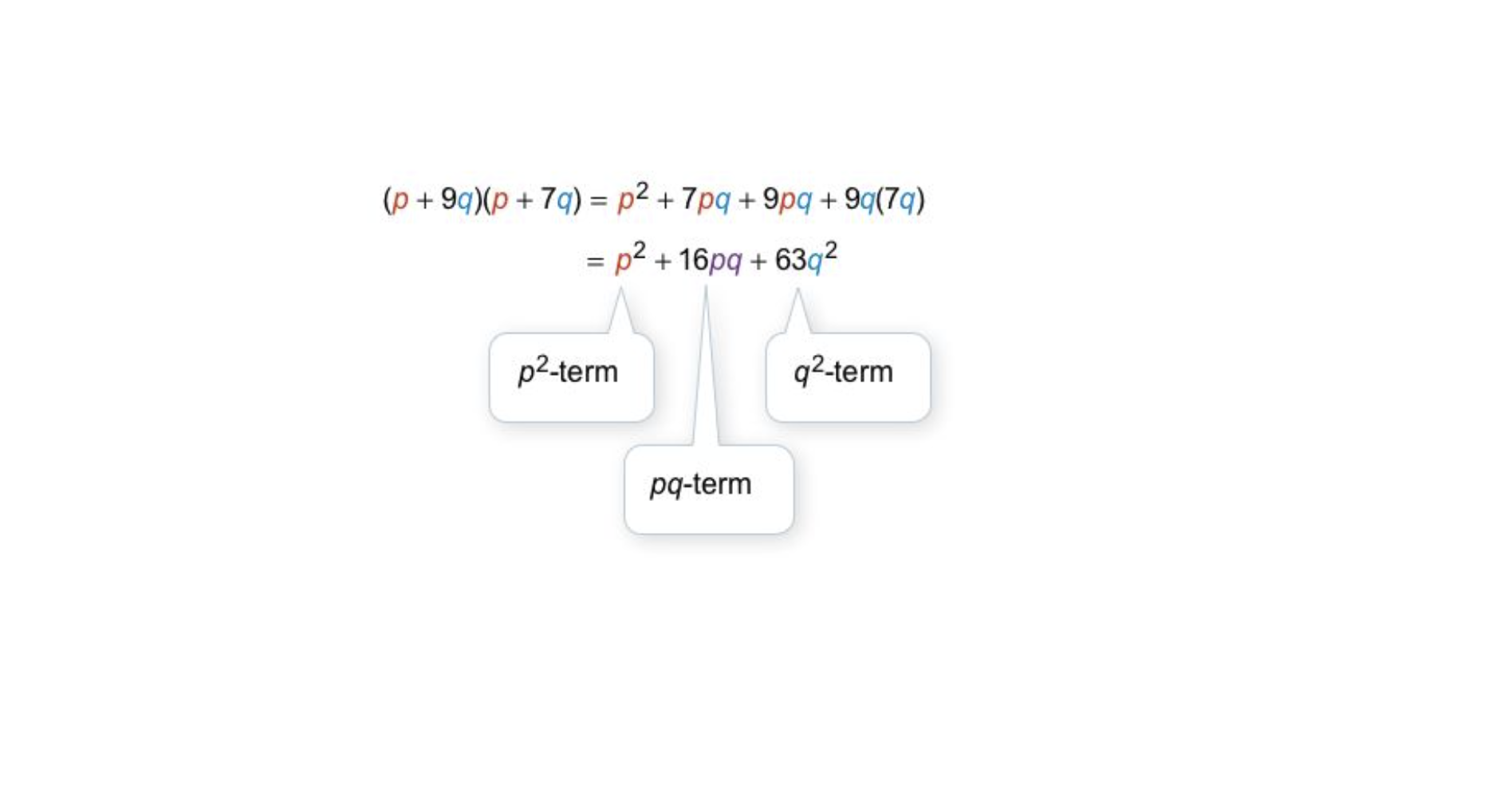zoom in8
10 pts
Problem 5 Got It?
A
B
C
Dzoom in9
10 pts
A
B
C
D
zoom in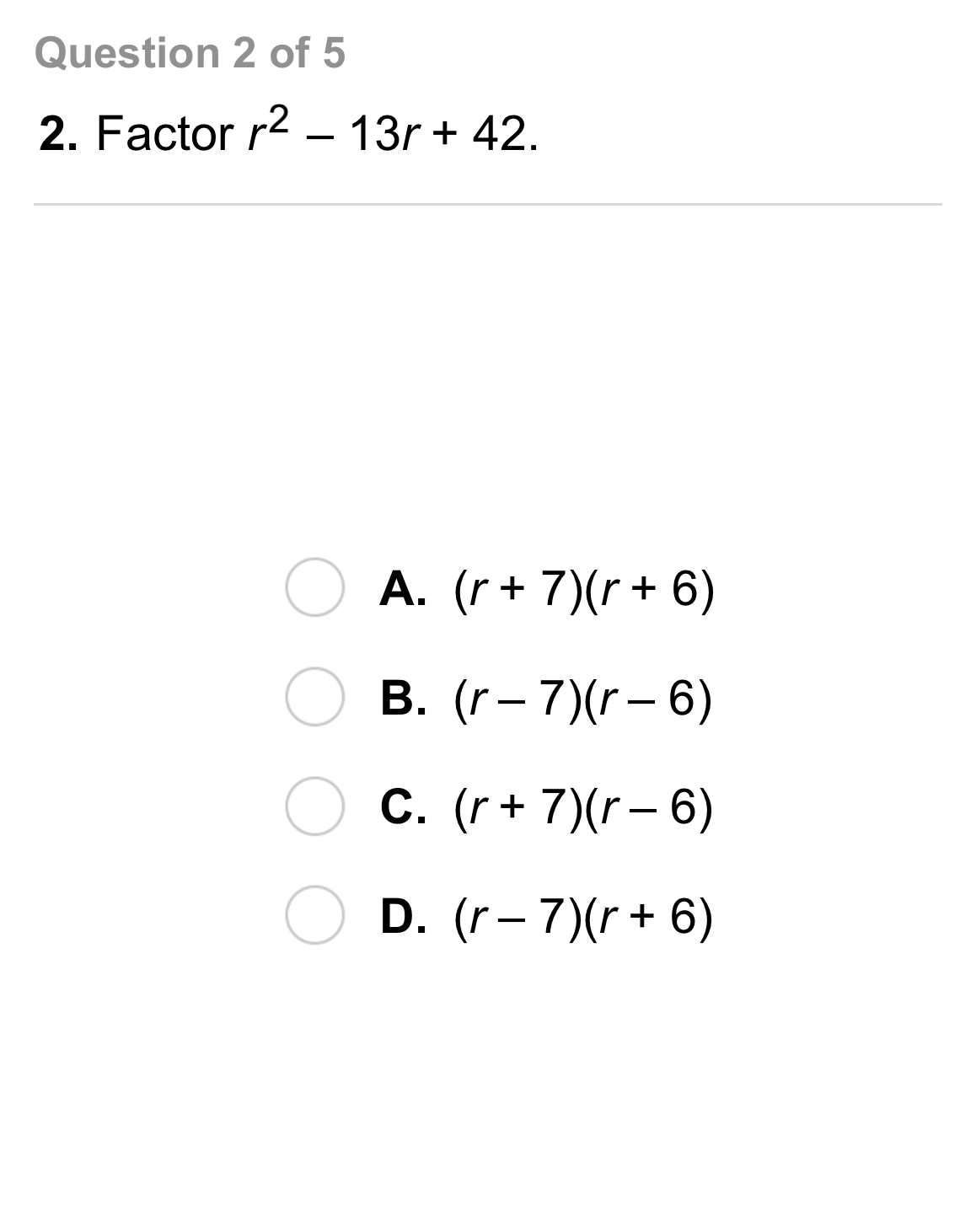10
10 pts
A
B
C
D
zoom in11
10 pts
A
B
C
D
zoom in12
10 pts
A
B
C
D
zoom in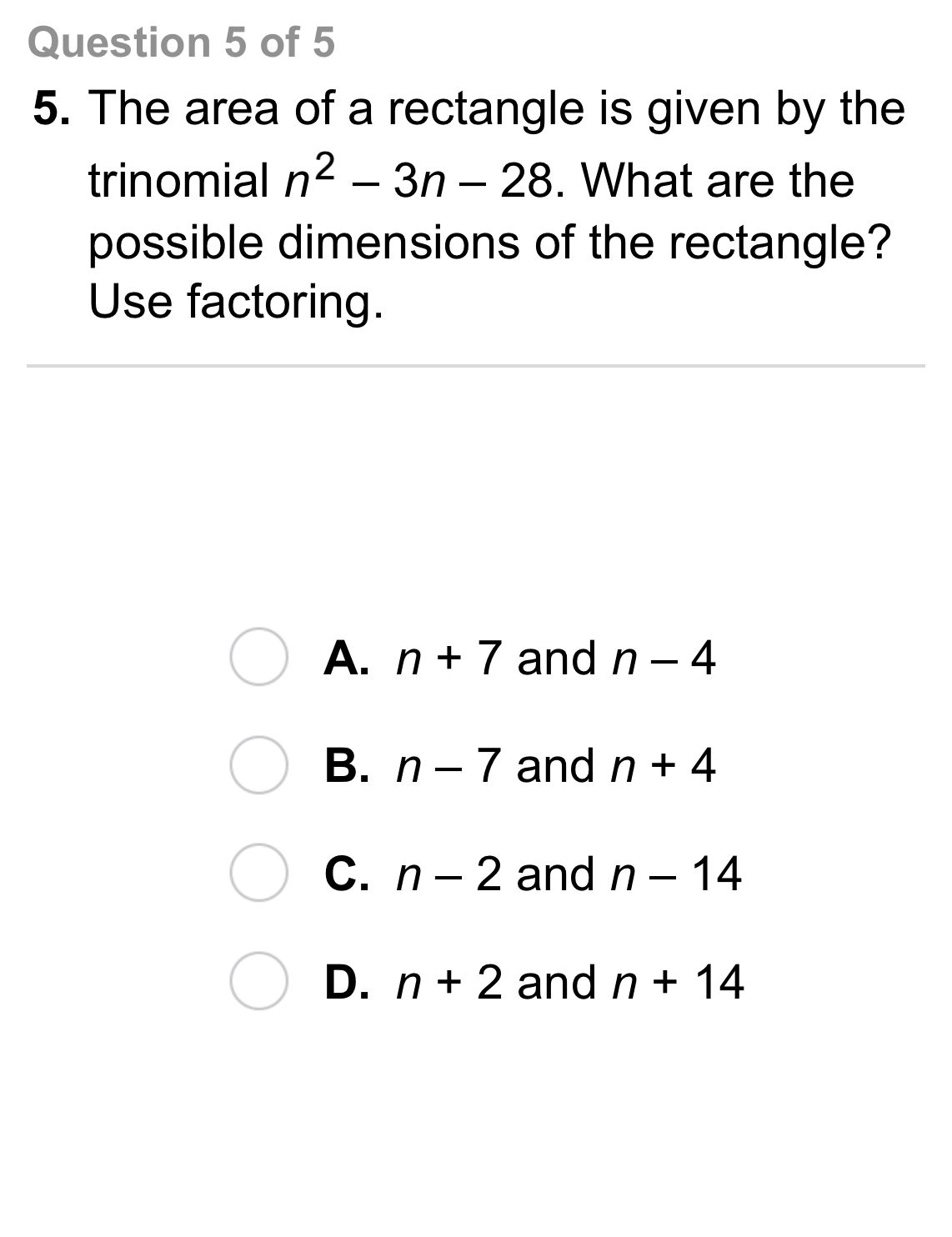13
10 pts
A
B
C
D14
5 pts
Analysis: Tell whether the sum of the factors of the constant terms should be positive or negative when you factor the trinomail.

positive
negative
15
5 pts
Analysis: Tell whether the sum of the factors of the constant terms should be positive or negative when you factor the trinomail.

positive
negative
16
5 pts
Analysis: Tell whether the sum of the factors of the constant terms should be positive or negative when you factor the trinomail.

positive
negative
17
10 pts
Reasoning: Under what circumstances should you look at pairs of negative factors of the constant term when factoring a trinomial of the form shown?
Consider positive and negative values of b and c.18
10 pts
Review Lesson 8-4: Simplify the product.

19
10 pts
Review Lesson 8-4: Simplify the product.

20
10 pts
Review Lesson 8-4: Simplify the product.

21
10 pts
Review Lesson 2-5: Solve each equation for x.
• x = b/a + d
Answer by dragging items here
• x = ad - b
Answer by dragging items here
• x = ad ÷ b
Answer by dragging items here
22
10 pts
Review Lesson 8-2: What is the GCF of the polynomial? Enter only a monomial.

23
10 pts
Review Lesson 8-2: What is the GCF of the polynomial? Enter only a monomial.

24
10 pts
Review Lesson 8-2: What is the GCF of the polynomial? Enter only a monomial.25
10 pts
Vocabulary Review: Use the Distributive Property. Match equivalent expressions.
• y³ + 3y² - 6y
Answer by dragging items here
• -y - 2
Answer by dragging items here
• y² + 2y
Answer by dragging items here
• 2y(y + 2) - 1(y + 2)
Answer by dragging items here26
10 pts
Use Your Vocabulary: Identify the trinomials.
• Trinomials
Answer by dragging items here
27
10 pts
Reflection: Math Success
Add to my formatives list